Profiler - Final Examination Multiple Choice Questions Flashcards Preview

Economics > Profiler - Final Examination Multiple Choice Questions > Flashcards

Flashcards in Profiler - Final Examination Multiple Choice Questions Deck (30):
1

The Chancellor has announced that the \$100m tax decrease will be financed by a \$100m decrease in government expenditure. What impact will these two actions have on national income?A. None; the effects of one will cancel out the effects of the other B. National income will be \$100m lower than it otherwise would be C. National income will be \$100m higher than it otherwise would be D. National income will be \$75m × balanced budget multiplier higher than it otherwise would be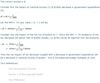2

3

In an economy where taxes equal \$40m, exports, government expenditure and investment expenditure are equal to each other and each equal to \$200m. Households consume 75% of their disposable income. Imports equal 25% of national income. What is the equilibrium level of consumption expenditure?A. \$825m B. \$865m C. \$925m D. \$1140m4

5

6

7

Investment expenditures were 0i2 before the marginal efficiency of investment schedule (MEI) shifted from I1 to I2. After the shift investment expenditures remained at 0i2.Notation: I1 is the investment level 1; I2 is the investment level for period 2.What could have caused investment expenditure to remain static?A. An increase in the rate of interest B. An increase in the ratio exports/GNP C. An increase in the value of the accelerator D. An increase in the unemployment rate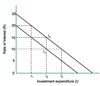8

9

Despite the existence of a budget deficit the government kept its election pledge and increased spending on hospitals and education. This extra government spending had no impact on national income, however, because there was 100% crowding out. What did occur however was the rate of interest increased the budget deficit increased investment and/or consumption expenditure decreased Which of the following is correct?A. I only B. II only C. II and III only D. I, II and III

The correct answer is D.100% crowding out means that the increase in government expenditure was matched by an equal decrease in consumption/investment expenditure caused by an increase in the rate of interest as shown below. Thus I is true.Given Y≡C + I + G and there were no changes in Y after the increase in G then C and/or I had to decrease. Thus III is true.Since a budget deficit existed before the increase in government expenditure and since there was no change in tax rates or Y then government revenues could not increase but expenditures did. Thus the deficit would increase. Thus II is true.Text References: The Relation Between the Unemployment Rate (U), Potential Output (Q) and Actual Output (Y) The Government Sector The Development of Macroeconomic Models Introduction Causes and Effects of Inflation Anti-Inflationary Policies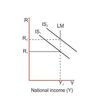10

11

12

13

14

15

16

From the above data, in index form, representing an economy, it can be inferred that between years t and t + 1 there was deflation overfull employment a decrease in labour productivity Which of the following is correct?A. I only B. I and III only C. II and III only D. I, II and III17

Which of the following points taken together indicate decreasing returns to the variable factor input capital?A. U and X B. U and V C. U and W D. U and Z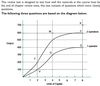18

Which of the following points taken together indicate constant returns to scale?A. U and X B. U and V C. U and Y D. U and Z19

Which of the following points taken together indicate constant returns to the fixed factor input, labour?A. V and T B. V and U C. V and W D. V and Z20

In response to student requests the university announced that 10% of next year’s students fees would be allocated to the construction of sports facilities. The sum in question was \$8 000 000 (\$8 million). The students were asked to reveal their preferences. The votes were:{image}What is the opportunity cost to the students of the swimming pool?A. \$8m B. A reduction in student fees C. The racquets centre D. The racquets centre plus the playing fields21

Short run profit maximising (loss minimising) behaviour requires that the firm produce which of the following weekly outputs?A. Zero B. Ow C. Ox D. Oy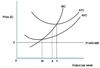22

23

24

What short-run loss will the profit maximising (loss minimising) firm make?A. (AR − AVC) × 0y B. (ATC − AR) × 0x C. (AR − AVC) × 0x D. (ATC − AR) × 0y25

The firm remains in business because its fixed costs are covered and some revenue remains to contribute to variable costs in the long run ATC will fall until it is tangential to the p = AR = MR line. Which of the following is correct?A. I only B. II only C. Both I and II D. Neither I nor II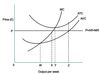26

The diagram shows for a profit maximising monopolist the marginal revenue curve (MR) derived from the demand curve facing the monopolist and the monopolist’s marginal cost (MC) and average fixed cost (AFC) curves.The profit maximising output is 20 units per week, i.e. where MR = MC.What price will the monopolist charge?A. \$10 B. \$15 C. \$20 D. The answer cannot be derived from the diagram and information given27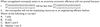2829

30

Trade in goods and services between Germany and France is balanced. It is predicted however that the French franc will appreciate significantly against the German mark and average German prices which had been increasing at 5% per annum will increase at 1% per annum while French price increases will remain constant at 6% per annum. Assuming these predictions come true how will this news be greeted by French and German exporting firms?